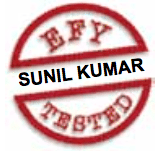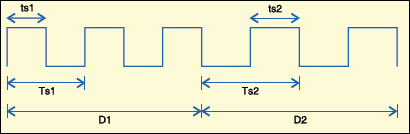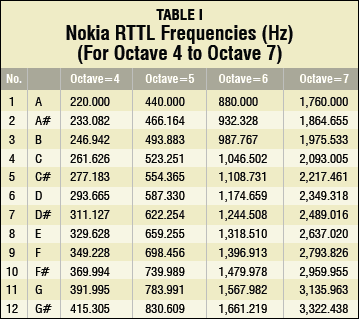Thursday, August 11, 2022

# Microcontroller-Based Ring Tone Player

This circuit for a ring tone player uses a AT89C51 microcontroller and ring tone text transfer language (RTTTL) to generate ring tones. -- By Subhajit RoyMobile phone ring tones sound like real audio recordings. It’s not because of the way the melodies are composed, but the protocol behind playing the melody. The ring tone text transfer language (RTTTL) is behind those wonderful lullabies and songs you have on your mobile phone.

Basically, a ring tone is the sound made by a mobile phone to indicate an incoming call or text message. Here we present a microcontroller-based ring tone generator.

The basics

The lowest resonant frequency of a vibrating object is called its fundamental frequency. Most vibrating objects have more than one resonant frequency and those used in musical instruments typically vibrate at harmonics of the fundamental. A harmonic is defined as an integer multiple of the fundamental frequency.

A cylindrical air column with both ends open vibrates with a fundamental frequency. Each end of the column must be an antinode, with one node at the centre for the air motion. Therefore if ‘λ’ is the wavelength of the sound produced by an open cylindrical air column, its length ‘L1’ will be:

L1 = λ/2 [(λ/4) + (λ/4 )]

So λ =2L1

Frequency n1 = V/λ (where ‘V’ is the velocity of sound)

By putting the value of ‘λ,’ we get:

n1 = V/2L1

If length is half, then:

Frequency n2 = V/L1

n1/n2 = (V/2L1) × (L1/V) = 1/2

or n1 = 2n2Fig.2: PWM signal, where Ts1, ts1 and D1 are the time period, half time period and duration of note 1, while Ts2, ts2 and D2 are the time period, halftime period and duration of note 2

Thus halving the length doubles the frequency, i.e., frequency n1 is created with one octave higher (2n1). If the length is made quarter of the original, the frequency becomes 4n1, i.e., two octaves higher.

If n1 is octave 1, then n2 is octave 2, then n3 is octave 3, and so on.

If the length is doubled, the frequency is halved. That is, the frequency becomes n1/2, which is one octave lower.

In music, frequency n1 is called a note. The pitch of a piano key or guitar string is described by the note.

According to musical frequency conventions, there are twelve notes in all, namely, A, A#, B, C, C#, D, D#, E, F, F#, G and G#, where ‘# ‘sign indicates a sharp note.

According to Nokia RTTTL specifications, note A with octave 5 has a frequency of 440 Hz (refer Table I):A5 = 440 Hz

So we get:
A6 = 880 Hz (A5x2)
A7 = 1760 Hz (A6x2)

A7 = 1760 Hz (A6x2)

The space between two consecutive octaves like A5 and A6 is divided into eleven equally spaced parts on the logarithmic scale. Thus there are twelve equally spaced notes per octave: A5, A#5, B5, C5, C#5, D5, D#5, E5, F5, F#5, G5, G#5 and then A6 starts.

RTTTL is a simple text-based format that you can use to create ring tones. An RTTTL file is made up of a single string divided into three sections separated by colons (:).

In the example of a Happy Birthday song given below:

d=4, o=5, b=125:16c, 32p, 32c, 32p, 8d, 32p, 8c, 32p, 8f, 32p, e, 16p, 16c, 32p, 32c, 32p, 8d, 32p, 8c, 32p, 8g, 32p, f, 8p, 16c, 32p, 32c, 32p, 8c6, 32p, 8a, 32p, 8f, 32p, 8e, 32p, 8d, 32p, 16a#, 32p, 32a#, 32p, 8a, 32p, 8f, 32p, 8g, 32p

1. The first section is the name of the RTTTL melody, i.e., ‘Happy Birthday Song.’

2. The second section defines the default values for the file. There are three categories of default values: duration (d=4), octave (o=5) and beat per minute (b=125).

3. The third section describes the melody.

It is a set of notes separated by a comma. The notes are given in the following format:

Duration (dn)   Note   Octave

8                    c         6

where ‘dn’ is the duration of the present note.

If either the duration (dn) or octave is not specified for a particular note, the default values are assumed.

After getting the notes, you must calculate the duration for which a note is to be played:

Number of notes per second to be played (N) = 60/beats per minute (b) =60/125

So to play music, all you need to do is to get the RTTTL ring tone of the particular music, then read its note and generate the frequency for the calculated duration (D).

“If the note is dotted; for example, ‘2b’ (here ‘b’ denotes note, not beats per second), the duration is made 1.5 times, so that D=1.5D.”

Previous articlePut Coin And Draw Power

#### 1 COMMENT

1.Lalu

How to find duration D?